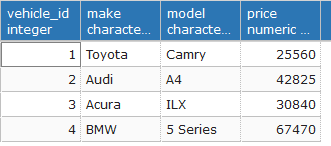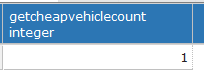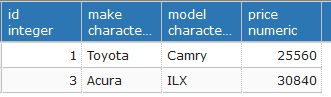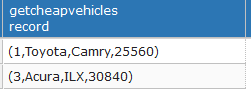### Development notes

Thoughts, notes and ideas about development

# PostgreSQL functions

PostgreSQL has a lot of built-in functions, such as Trigonometric Functions, String Functions, IP address functions, etc. But we’re not limited by built-in functions. PostgreSQL (as well as other RDBMS) allows creating custom functions. In this tutorial we will take a look at user-defined functions.

PostgreSQL supports the following programming languages for writing functions:

• SQL
• PL/pgSQL
• C
• PL/Python
• PL/Tcl
• some other languages

### Create functions with PL/pgSQL

Functions written in PL/pgSQL can contain a variable declaration, conditional and looping constructions, exception handling and so on. PL/pgSQL is a block-structured and case-insensitive language.

Base syntax for creating functions in PostgreSQL looks into the following way:

``````CREATE
OR REPLACE FUNCTION function_name (arguments)
RETURNS r_type AS \$\$
DECLARE
declarations
BEGIN
statements
END;
\$\$
LANGUAGE plpgsql;
``````

`CREATE OR REPLACE FUNCTION` - creates a new function named by `function_name` in case when such function doesn’t exists, otherwise replaces the existing function.
`arguments` - is a list of function’s arguments. Functions can have zero or more parameters(arguments). We may specify only parameter type, e.g. `(int4, varchar(40))`. In that case within a function body, the parameters of the function are referred to as `\$1, \$2,..` and so on, in the order they are defined. But we also can specify parameters names together with its types `(price int4, name varchar(40))`.
`RETURNS` - returned data type from the function. In our case `r_type` is specified type which will be returned from the function.
`DECLARE declarations;` - is a declaration section, which contains the variables names and its data types.
`BEGIN Statement;` - is a statement section which is the main body of the function. It may contain unlimited nested sub-blocks ( with DECLARE, BEGIN, END sections).
`END` - finishes the statement block.

`BEGIN/END` sections doesn’t start or stop transactions. It’s used for grouping blocks of code.

### Function example

Let’s assume we have a table `vehicle`which has the following columns:

``````vehicle_id - vehicle's ID
make - vehicle's make
model - vehicle's model
price - vehicle's price in \$
``````

Now let’s create a function which accepts desired price as a parameter and prints the amount of vehicles with price lower than or equals to desired price:

``````CREATE
OR REPLACE FUNCTION getCheapVehicleCount(desiredPrice INTEGER)
RETURNS INTEGER AS \$\$
DECLARE
total INTEGER;
BEGIN
SELECT count(*)
INTO total
FROM vehicle v
WHERE v.price <= desiredPrice;
RETURN total;
END;
\$\$
LANGUAGE plpgsql;
``````

Now let’s create a simple table `vehicle`:

``````DROP TABLE IF EXISTS vehicle;

CREATE TABLE vehicle
(
vehicle_id SERIAL       NOT NULL,
make       VARCHAR(100) NOT NULL,
model      VARCHAR(100) NOT NULL,
price      NUMERIC(5)   NOT NULL
);
``````

and fill in it with the test data:

``````INSERT INTO vehicle (make, model, price)
VALUES ('Toyota', 'Camry', 25560);
INSERT INTO vehicle (make, model, price)
VALUES ('Audi', 'A4', 42825);
INSERT INTO vehicle (make, model, price)
VALUES ('Acura', 'ILX', 30840);
INSERT INTO vehicle (make, model, price)
VALUES ('BMW', '5 Series ', 67470);
``````

### Verifying function

For verification let’s find how many vehicles have price less or equals to 30000\$:

``````SELECT getCheapVehicleCount(30000);
``````

The output will look into the following way:### Return multiple rows from the function

Let’s assume we need a function which returns a list of vehicles that have price lower than or equals to desired price. Such function will look into the following way:

``````CREATE
OR REPLACE FUNCTION getCheapVehicles(desiredPrice INTEGER)
RETURNS TABLE (id int, make VARCHAR(100), model VARCHAR(100), price NUMERIC(5))  AS \$\$

BEGIN
RETURN QUERY SELECT * FROM vehicle v WHERE v.price <= desiredPrice;
END;
\$\$
LANGUAGE plpgsql;
``````

If we call the function `getCheapVehicles` into the following way:

``````SELECT *
FROM getCheapVehicles(40000);
``````

We’ll get the following output:In case when we call `getCheapVehicles` function without `* FROM` we’ll get the following output:### PERFORM

When we call a function which doesn’t return any result or we don’t need the returned result we may discard result by using `PERFORM` keyword:

``````PERFORM
query;
``````

More detailed information about functions in PostgreSQL could be found in official documentation here: CREATE FUNCTION.

comments powered by Disqus